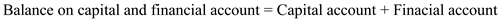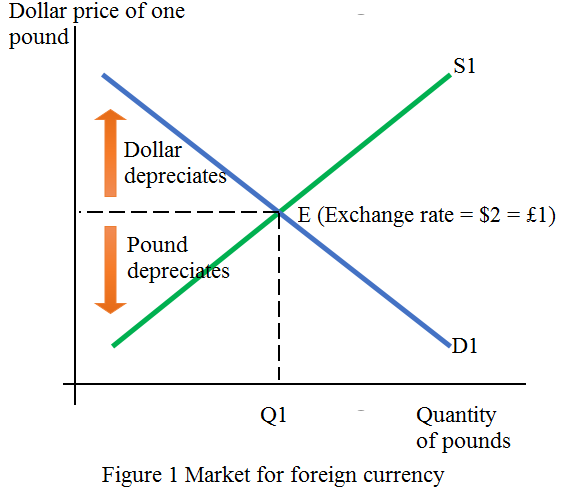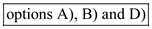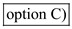(a) We can find the balance on goods by simply taking the values of the goods exported and the goods imported. Given: • Goods-exported is \$40 billion, and • Goods-imported is \$30 billion. Thus,Therefore, balance of goods amount to \$10 billion. (b) To know the balance of goods and services is you need to first sum up the total exports and imports i.e. simply take the value of goods and services exported and imported. Given that: • Value of imported goods, or \$30 billion, and • Value of imported services, or \$10 billion. Thus,• Value of goods exported is \$40 billion and • Value of services exported is \$15. Thus,Now to calculate the balance on goods and services we need to deduct total imports from total exports as under:Therefore, the balance on goods and services is \$15 billion. (c) The balance on the current account includes both the balance for goods and services, net investment income, and net transfers. Given that: • Net investment income is \$5 billion and • Net transfers are \$10 billion. • Balance for goods and services is \$15 billion (calculated in part b) Thus,Therefore, current account balance is \$20 billion , which means that there is surplus in current account. (d) The balance on capital and financial account is equal to the sum of the balance on the capital account (which is zero) and the sum of the balance on the financial account given as under:As the balance on capital account is zero, you need to first calculate the balance on financial account.Therefore, the balance on capital and financial account is \$20 billion. Sale of official reserves abroad counts as credit for the balance of payments statement of the country, and more specifically, it is an increase of foreign purchases of domestic assets. Without this sale, you can observe that the capital and financial account will have \$10 billion less, which means that the capital and financial account will be \$30 billion. Remember that the current account is at \$20 billion. Therefore, this shows that the domestic country has a balance-of-payments deficit.
Foreign exchange price of a given currency is determined by the demand function and the supply function of the given currency in terms of the foreign currency. The demand function is usual downward sloping while the supply function is sloping upwards. Together they determine the price of one currency in terms of given foreign currency and its quantity demanded and supplied. The dollar price of pound is established at \$2 for each pound in Figure 1. The demand curve D1 and supply curve S1 meet at point E at which the foreign exchange rate of pound is determined.In case there is an appreciation of dollar, the same dollar would now buy more pounds. This implies that the price of pound would fall, leading to its depreciation. Figure 1 suggests that when pound depreciates, the quantity of pounds demanded rises. With higher dollar price of pound, the dollar would depreciate. Figure 1 suggests the supply function slopes upwards, implying that when dollar price of pound rises, the quantity of pounds supplied increases. Also, when the demand for pound increases, the demand curve would shift right, resulting in dollar depreciation and relative pound appreciation. Hence,are incorrect. Point E in Figure 1 shows that the equilibrium exchange rate is \$2 for 1 pound. This in turn, also implies that the same value in terms of pounds is £0.5 for each dollar. Hence,is correct.